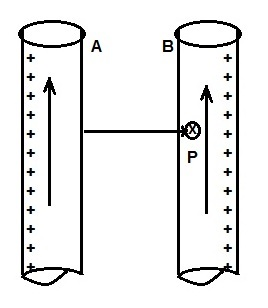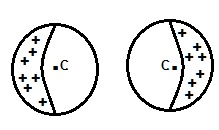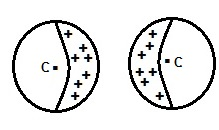# Proximity Effect – Definition, Cause and Affecting Factors

### Definition:

Proximity Effect is the phenomena of non-uniform current distribution on the surface of adjacent current carrying conductor due to the effect of another current carrying conductor in its proximity. Since in cables, the conductors are very near to each other, this effect is dominant. Whereas in overhead lines as lines are far apart when compared with cable, this effect can be neglected.

### Cause of Proximity Effect:

The main reason for proximity effect is production of magnetic field in the surrounding of a current carrying conductor. We know that any current carrying conductor produces magnetic field in its surrounding as per Biot Savart Law. When this magnetic field links with the adjacent conductor, it gives rise to a circulating or eddy current in it. Because of this eddy current, the current distribution on the nearby becomes non-uniform. This leads to additional resistance to the flow of current in the nearby conductor. Hence due to proximity effect, the net ac resistance of conductor increases. To better understand the proximity effect, let us consider the figure below.The above figure shows two conductors carrying current in the same direction. The magnetic field created by conductor A will link with conductor B. Similarly, the magnetic field created by conductor B will link with conductor A.

The direction of magnetic field at any point P inside the conductor B will in going inside the plane of device in which you are reading this article. Since current is flowing in upward direction, this means that free electrons are moving in downward direction. The direction of force on the free electrons due to the magnetic field of conductor A will be given by the cross vector product of velocity vector of electron and magnetic field vector at point P. From the cross vector product, the free electrons of conductor B will be drifted toward the inner surface i.e. toward conductor A and hence the current will flow mostly in the farthest surface of conductor B.

The same reasoning when applied to conductor A, it can be seen that current in the conductor A will mostly flow in the farthest surface. This effect of re-distribution of current on the conductor surface is called the proximity effect. This is so called proximity effect as this is due to the effect of proximity (nearby) conductor.

To summarize, when two current conductors carry current in the same direction, the current gets distributed to the farthest half of both the conductor. This is shown in figure below.Similarly when two conductors carry current in opposite direction, the current in conductors gets concentrated toward the inner facing surface of both the conductors as shown in figure below.### Factors affecting Proximity Effect:

Following are the factors on which proximity effect depends on:

• This effect increases with increase in frequency. This is the reason, this effect is not observed in case of DC. In case of DC, as the current is uniformly distributed over the entire cross-section of conductor, the magnetic force on the free electrons inside conductor is less prominent. Hence this effect is not observed.
• More the diameter of conductor, the more will be this effect.
• This effect also depends on the material of conductor. If the material is of ferromagnetic nature, proximity effect will be more.
• Proximity effect is more in case of solid conductor. This is the reason, stranded or ACSR conductors are used to reduce this effect.

### One thought on “Proximity Effect – Definition, Cause and Affecting Factors”

1.Jason S. says:

The effect should be observed in DC too.

This site uses Akismet to reduce spam. Learn how your comment data is processed.